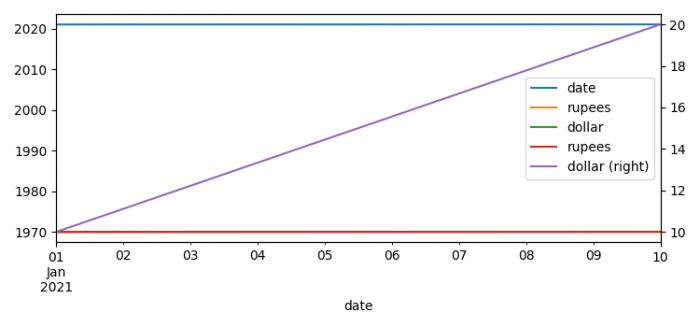# Plot multiple time-series DataFrames into a single plot using Pandas (Matplotlib)

To plot multiple time-series data frames into a single plot using Pandas, we can take the following steps −

• Set the figure size and adjust the padding between and around the subplots.
• Create a Pandas data frame with time series.
• Set the time series index for plot.
• Plot rupees and dollor on the plot.
• To display the figure, use show() method.

## Example

import numpy as np
import pandas as pd
from matplotlib import pyplot as plt, dates

plt.rcParams["figure.figsize"] = [7.50, 3.50]
plt.rcParams["figure.autolayout"] = True

df = pd.DataFrame(dict(date=list(pd.date_range("2021-01-01", periods=10)), rupees=np.linspace(1, 10, 10), dollar=np.linspace(10, 20, 10)))

df.set_index(pd.to_datetime(df.date), drop=True).plot()
df = df.set_index(pd.to_datetime(df.date), drop=True)
df.rupees.plot(grid=True, label="rupees", legend=True)
df.dollar.plot(secondary_y=True, label="dollar", legend=True)

plt.show()

## Output